Important questions on Electric Charge for JEE advanced and Main

In this page we have Important questions on Electric Charge for JEE advanced and Main . Hope you like them and do not forget to like , social share and comment at the end of the page.

Question 1
Two point charges q1 and q2 are located with points having position vectors r1 and r2 .
1. Find the position vector r3 where the third charge q3 should be placed so that force acting on each of the three charges would be equal to zero.
2. Find the amount of charge q3
Question 2
Consider a thin wire ring of radius R and carrying uniform charge density λ per unit length.
1. Find the magnitude of electric field strength on the axis of the ring as a function of distance x from its centre.
2. What would be the form of electric field function for x>>R.
3. Find the magnitude of maximum strength of electric field.
Question 3
Two equally charged metal balls each of mass m Kg are suspended from the same point by two insulated threads of length l m long. At equilibrium, as a result of mutual separation between balls, balls are separated by x m. Determine the charge on each ball.
Question 4
There are two identical particles each of mass m and carrying charge Q. Initially one of them is at rest and another charge moves with velocity v directly towards the particle at rest. Find the distance of closest approach.
Question 5
Find the electric field at the centre of uniformly charged semi circular arc having linear charge density λ.
Question 6
Two opposite corners of square carry charge –q and other tow opposite corners of same square carry charge +q as shown below in the figure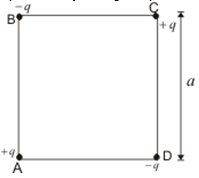All the four charges are equal in magnitude. Find the magnitude and direction of force on the charge on the upper right corner by the other three charges.
Question 7
A rigid insulated wire frame in form of a right angled triangle ABC is set in vertical plane as shown below in the figure.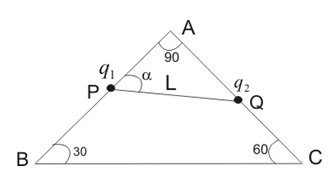Two beads of equal masses m and each carrying charges q1 and q2 are connected by a chord of length l and can slide without friction on the wires. Considering the case when beads are stationary determine
1. the normal reaction on the beads
2. the angle α
3. tension in the chord
If the chord is now cut what are the value of charges for which beads continue to remain stationary.
Question 8
Inside a ball charged uniformly with volume density ρ , there is a spherical cavity. The centre of cavity is displaced with respect to the centre of the ball by a distance a. Find the field strength inside the cavity assuming the permittivity to be equal to unity.
Question 9
An electric dipole is placed at a distance x from a infinitely long rod of linear charge density λ.
1. Find the net amount of force acting on the dipole.
2. Assuming that dipole is fixed at its centre find its time period of oscillations if the dipole is slightly rotated about its equilibrium position.
Question 10
An electric charge Q is uniformly distributed over the surface of a sphere of radius R. Show that the force on a small charge element dq is radially outwards and is given by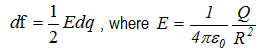is the electric field at the surface of the sphere.
Question 11
A thin fixed ring of radius R has a positive charge of +q C uniformly distributed over it. A particle of mass m and charge –q is placed on axis at a distance x from the centre of the ring. Show that the motion of negatively charged particle is approximately simple harmonic.
Question 12
Consider the figure given below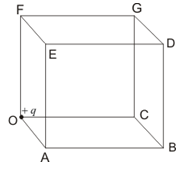A positive charge +q is placed at corner of the cube. Find the electric flux through the right face BCGDB of the cube.

Question 13
Consider a sphere of radius r having charge q C distributed uniformly over the sphere. This sphere is now covered with a hollow conducting sphere of radius R>r.
1. Find the electric field at point P away from the centre O of the sphere such that r<OP<R.
2. Find the surface charge density on the outer surface of the hollow sphere if charge q’ C is placed on the hollow sphere.
Question 14
1. Find the electric field inside the uniformly charged sphere of radius R and volume charge density ρ using Gauss’s law.
2. Use Gauss’s law to find the electric field outside, at a point on the surface and at any point inside a spherical shell of radius R, carrying a uniform surface charge density σ.
Question 15
(a) Show that the normal component of electrostatic field has a discontinuity from one side of a charged surface to another given by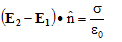Where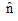a unit vector is normal to the surface at a point and σ is the surface charge density at that point. (The direction of is from side 1 to side 2.)
(b) Show that the tangential component of electrostatic field is continuous from one side of a charged surface to another.
Question 16
Consider a cylinder as given below in the figure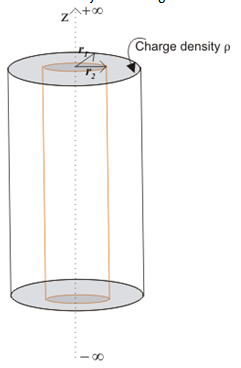Volume between radius r1 and r2 contains uniform charge density ρ C/m3. Use Gauss’s law to find electric field in all regions.

Solutions